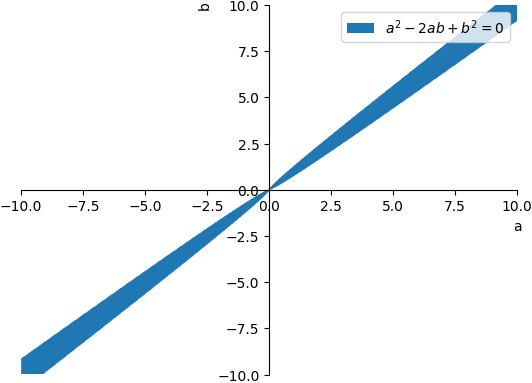MathsGee is Zero-Rated (You do not need data to access) on: Telkom |Dimension Data | Rain | MWEB

2 like 0 dislike
31 views
If $a+b: \sqrt{a b} = 2: 1$ then what is $a:b$?
| 31 views

0 like 0 dislike
If $a+b: \sqrt{a b} = 2: 1$ then what is $a:b$?

We can form the equation $a+b=2(\sqrt{ab})$ given the ratio of $2:1$

Simplifying gives:

$$a+b=2(\sqrt{ab})$$

$$\dfrac{a+b}{2}=\sqrt{ab}$$

$$\left(\dfrac{a+b}{2}\right)^2=ab$$

$$\dfrac{(a+b)(a+b)}{4} = ab$$

$$(a+b)(a+b) =4ab$$

$$a^2+2ab+b^2 = 4ab$$

$$a^2+2ab+b^2 - 4ab =0$$

$$a^2-2ab+b^2 =0$$

Solution
$\left\{ \begin{array} {l} \,a = b \\\, b = a \end{array} \right.$

Simplification
$a^{2} - 2 a b + b^{2} = 0$

Graphby Bronze Status (9,598 points)

1 like 0 dislike
0 like 0 dislike
0 like 0 dislike
1 like 0 dislike